## Indicators

•Cited by SciELO
•Access statistics

•Cited by Google
•Similars in SciELO
•Similars in Google

## Print version ISSN 0012-7353

### Dyna rev.fac.nac.minas vol.79 no.176 Medellín Nov./Dec. 2012

REGISTRATION OF RANGE IMAGES USING A HISTOGRAM BASED METRIC

REGISTRO DE IMÁGENES DE RANGO MEDIANTE UNA METRICA BASADA EN HISTOGRAMA

M.Sc Departamento de Ciencias de la Computación y de la Decisión, Universidad Nacional de Colombia - Sede Medellín, Colombia, amaldona@gmail.com

GERMAN SÁNCHEZ TORRES
Ph.D Universidad del Magdalena - Santa Marta, Colombia, gsanchez@unimagdalena.edu.co

JOHN WILLIAN BRANCH
Ph.D Departamento de Ciencias de la Computación y de la Decisión, Universidad Nacional de Colombia - Sede Medellín, Colombia, jwbranch@unal.edu.co

Received for review July 25th, 2012, accepted October 9th, 2012, final version November, 2th, 2012

ABSTRACT: In this paper, a new approach for coarse registration of triangular meshes for three-dimensional object reconstruction is proposed by using the shape-index measure for topological description and the Earth Mover's Distance (EMD) metric for region similarity estimation. Two of its main advantages are independence from initial pre-alignment and spatial transformation, obtaining a more accurate registration regardless of the initial viewing position.

KEYWORDS: Range Image registration, 3-D reconstruction, shape index, EMD.

RESUMEN: En este trabajo se propone un método de registro grueso de mallas triangulares para la reconstrucción de objetos tridimensionales, empleando el índice de forma para obtener información topológica de la superficie y la comparación de regiones con forma similar empleando la métrica Earth Mover's Distance (EMD). Las principales ventajas de este método es que permite independencia de la pre-alineación inicial y transformación espacial, permitiendo obtener un registro adecuado sin importar la posición inicial de cada vista.

PALABRAS CLAVE: Registro de imágenes de rango, reconstrucción 3-D, índice de forma, EMD.

1. INTRODUCTION

3D reconstruction is the process of generation of a computerized model from a digitalized object, keeping its physical features such as dimension, volume and shape . This process plays a prominent role in computer-aided design systems (CAD) due to its robustness regarding complex objects with arbitrary topology . The generation of these models has different application fields such as object recognition, facial reconstruction for identification and data exchange of archaeological findings.

The method proposed in this paper focuses on the registration stage. Problems raised in this stage include the non-alignment produced when taking two or more range images from different points of view and without any control of the relative positions between the object and the sensor. Once the acquisition stage is over by scanning the object's surface, and the visualization of the images in a unique system of coordinates is done, different overlapping views are obtained -without any coherence.

The aim of the registration is to transform all the acquired partial views to a common coordinate system. Because a simple range image can contain just the points of the scene that are visible to the sensor, it is necessary to link multiple range images in a single set of points to obtain the entire model of the scene.

Classification of registration methods is complex and it can be performed from different aspects. A common classification is done according to the precision level in which the method links both images. Thus, a coarse registration can exist as well as a fine one, whether the aim is to give an initial alignment or to link the images precisely.

This paper proposes a method of coarse registration of triangle mesh for three-dimensional object reconstruction, by using the shape-index measurement for the topological description of the surface. One of the most important advantages of this method is that it allows independence from initial pre-alignment and spatial transformation, making it possible to obtain an accurate registration regardless of the initial position of each view. The main difference regarding similar works lies on the fact that alignment is not based on analysis of pairs of points, like the classic model, but on regions. The matching of the regions is based on a similarity metric estimated using a histogram representing the geometric features of each region.

This paper is structured as follow: Section 2 presents relevant works of the state of the art. Section 3 describes the proposed method. Sections 4 and 5 show the obtained results and the conclusions, respectively.

2. RELATED WORKS

The main works in the state of the art are described below. The most used registration method to align pairs of images consists in the minimization of the difference between the set of points of each image, called Iterative Closest Point (ICP). Subsequently, an important number of works have followed this direction trying to improve different aspects related to the method, such as its efficiency, level of error, matching algorithm, among others. Additional works include those of Chua , who introduces the Point Signature method. This method is used to represent the structure of a specific neighborhood. For a point p and a sphere S centered at p with radius r, the method estimates the largest positive distance from a point p to the curve C. the curve C is a 3D curve formed by the intersection of S and the object surface. The correspondence between points is established between two points with similar Point Signatures. The matching process is fast but, sometimes, it requires interpolation, which affects quality; besides, calculating the intersection of the object's surface with the sphere is not easy. It is invariant to rotation and translation but it is sensitive to noise.

The Spin Image method, introduced by Johnson , is based on the creation of images called Spin Images, which locally represent the surface geometry with respect to a point, allowing characteristic zones on the surface to be obtained and to perform the registration with other surfaces. In general, this method is robust against noise but it depends of the resolution used (although, there are variations that make interpolations on every image), and it has some problems with symmetric images.

Chung and Lee  introduce the registration method Principal Component Analysis (PCA). Their aim is to reduce a wide set of variables to a new smaller set, without losing important parts of the original information. The direction of the main axis of the cluster of dots is used to align a sequence of range images. If the overlap region is large enough, the axes of the images must match so that the axes are aligned by applying just one transformation and therefore, the images are aligned too. This method implies the calculation of the co-variance matrix of each image, which implies high speed and precision with enough points.

DARCES (data-aligned rigidity-constrained exhaustive search) based on RANSAC (RANdom SAmple Consensus) was introduced by Chen et al. . It is based on the search for the best match of three points between two range images, in order to calculate the rigid transformation. The points (main, secondary and auxiliary ones) are characterized by the distance between them. The method is robust against atypical data and noise but can only be used when the amount of points in the images is small, because calculation time increases considerably. Tarel et al.  proposed the Algebraic Surface Model (ASM) method, which estimates a transformation between surfaces represented as polynomials estimated by the 3L Fitting method, which is a linear algorithm based on minimum squares. The matching method is faster, but it requires the estimation of the normal at each point; this can considerably increase the calculation time. However, it can bring good results with an overlap area lower than 15%.

Stamos and Leordeanu  propose a method calculating the match between images from straight lines. The algorithm was applied to structure registration with plane regions such as buildings, and it obtains good results, however the difficulty with this method lies in the fact that it is not automatic.

Registration using Genetic Algorithms (GA) was introduced by Brunnström and Stoddart , and consists in the search of an individual whose chromosome is composed of 6 parameters determining the rigid transformation that aligns a couple of images: 3 elements of the translation vector, and 3 rotation angles. Variations of this method are presented using different GA aptitude functions. This method is robust against noise, local minimum and atypical data, although as a result of evolutionary techniques, it requires a long calculation time and, depending on the aptitude function, the cost of the matching calculation can be very high. Feldmar and Ayache  propose a method based on the difference of points characterized by their main curvatures as a geometrical feature for the matching process. This method requires just one match in order to calculate the transform. It calculates curvatures (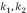) at a point, where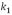and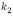are the maximum and the minimum curvature of the surface at the specific point. In addition, the normal vector and the direction of the main curvature are also considered. Even though it is not always possible to calculate curvatures and directions at a point, a parametrized surface is proposed (similar to Tarel's  proposal). The biggest problem of this method is that it is not robust; the algorithm stops when it finds a good match, presenting some false-positive.

Other works propose variants of some stages of the ICP classic method, such as , which proposes monitoring the sensor position, and the identification and indexing of surface features; or in , which propose using Minimum Variance Estimator (MVE) for the transform estimation; and , which propose using Least Median of Squares (LmedS), which aims to minimize the average of matching residuals.

One of the main weaknesses of registration methods lie with the algorithm executed when matching, that is, the stipulated metric to determine whether two points of different images constitute the same point, or the closest one, in the treated object. For this reason, the proposed approach is based on determining the matching of two points based on the geometry of the surrounding region.

3. REGISTRATION METHOD BASED ON GEOMETRIC FEATURES

The method proposed here consists of aligning the partial views from geometric features described by the shape-index measure. The process is carried out as follows:

1. The shape index is calculated for every vertex from the main curvatures values in each vertex.
2. Three vertexes are selected from the first partial view, and a histogram of shape-index of the neighborhood around each vertex is generated.
3. On the second view, a search for vertexes having similar histograms is done using a variant of the EMD (Earth's Mover Distance) method to calculate the distance between histograms. This search will generate a list of similar vertexes on the second view for each vertex selected on the first one.
4. By using the list of similar vertexes the aim is to find the match with less alignment error. Alignment is only made between the pairs formed by the vertexes selected from the first view, and their matches on the second view. That is why the matching search space is reduced.
5. The alignment of the complete views is done by using the pairs obtained in the previous step, and the alignment error, as well as the Surface Interpenetration Index, is calculated.

Next, each step is described.

3.1. Shape-index calculation
As mentioned before, in order to calculate the shape index it is necessary to calculate the main curvatures for each vertex. Curvature is an invariant measurement of a surface that indicates how much it is curved. They are said to be invariant because they remain constant against translations and rotations, although not for scale changes.

Curvature measures the tangent vector's change of direction in a curve. This vector corresponds to the reciprocal of the tangent sphere's ratio at the surface. It is obtained from the Second Fundamental Form, which represents the variation of the normal from point to point. For a continuous surface it applies: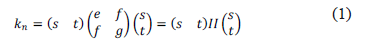to any vector (s, t) in the tangent plane (expressed in terms of an orthonormal coordinates system centered on the point). Matrix II is known as Weingarten matrix or Second Fundamental Form, which can be diagonalized to obtain: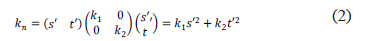The maximum and minimum values of normal curvature at a point p of the surface S are called principal curvatures  of S at p, denoted by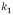and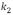. Vectors (s', t'), in which the extreme values are found, are called principal directions.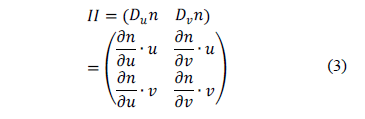where (u, v) are the directions of the orthonormal coordinates system at the point. Multiplying II by a vector of the tangent plane, the derivative of the normal in that direction is obtained: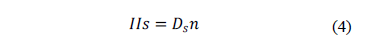Although this expression is defined for continuous surfaces, an approximation can be carried out for the discrete case using finite differences: for a triangle having the direction of its edges defined, we have the differences of the normal in these directions . This brings a set of linear restrictions for the calculation of II using least squares. Once the system is solved, we have the principal curvatures per vertex.

Once the curvatures are obtained, the associated shape index can be obtained. The shape index was proposed in , and it is an angular physics measurement that captures the intuitive notion locally at a surface's point: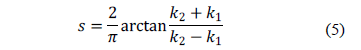The index range varies between -1 and 1. A point of a convex surface having the same principal curvatures has a shape index of 1. A point of a concave surface having the same principal curvatures has a shape index of -1. A saddle point having the same magnitude of principal curvatures but different signs has a shape index of 0.

3.2. Search for vertexes having histograms of similar shape
Once the shape indexes per vertex of each view are obtained, a) at least three vertexes from the first view are manually selected; b) a histogram is generated with the shapes indexes of the neighborhood surrounding each vertex, and c) a search for the vertexes from the second view having similar histograms is carried out.

A histogram represents the frequency of a variable's values. The quantized variable is categorical, that is, its values are alphanumeric categories or labels, and the quantity of possible values is finite. Every value represented in the histogram corresponds to a class (bin). In general, the quantity of classes in a histogram is the same as the quantity of values of the evaluated variable. But in this case, the shape index is a continuous variable, so it is not possible to represent it directly in a histogram; therefore, we group its possible values into sub-intervals. The interval [-1,1] was divided into 10 sub-intervals, each one associated with a histogram class.

Given two histograms, a quantitative measurement of their difference is required, reflecting the similarity perception as best as possible. Rubner and Guibas  propose the EarthMover'sDistance (EMD) metric to establish the difference between two distributions, which consists in the calculation of the minimal redistribution quantity needed to convert one distribution into the other. The EMD is calculated by using a linear optimization algorithm based on the solution to the transport problem  , which is a particular case of a linear programming problem in which the costs of supplying a series of demand points (destination), from a group of supply points (origin), must be minimized.

3.3. Similarity Calculation between Histograms
Given two histograms P, Q, EMD is defined as: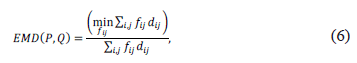Subject to: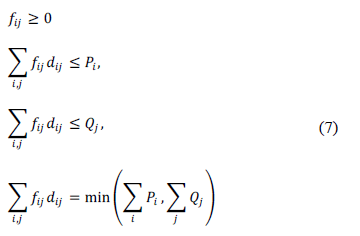where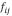denotes the network flows associated to transport problem,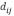represents the transported quantity from class i of P to class j of Q, andrepresents the ground distance between class i and class j. .

3.4. Error Calculation and Surfaces Interpenetration Measure
Surfaces Interpenetration Measure (SIM) is based on the natural effect resulting from surface registration, due to small local distortions caused by the limitations in the sensor's precision, or by noise .

A more accurate evaluation can be established by quantifying the interpenetration, and it is possible to offer a more robust control of the registration, even though in this work it is used just as a measurement for evaluating the registration's quality. A high SIM value represents a good registration, a bad alignment produce a low value.

After the alignment of two views, A and B, identification of interpenetration points in A with respect to B is carried out. We will call them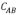. For each point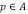a neighborhood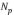of radius n centered on p is defined. Defining q as a point in the neighborhood, c as the corresponding point to p in B, obtained through a search of neighboring points by using a kd-tree , and y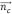the normal vector in c. Therefore, the set of interpenetration points in A is given by: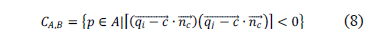where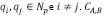correspond to points in A of neighborhoods which include at least a pair of points separated by a local tangent plane, obtained from corresponding points in B, as seen in Figure 1.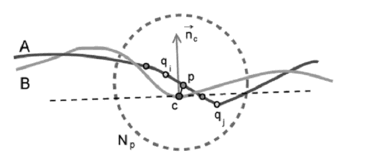3. RESULTS

To evaluate the method proposed here, the described steps were applied to pairs of views of the Stanford bunny and armadillo , and a pre-Columbian vase.

The first step consists in loading the triangular mesh of the views to be aligned,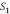and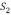, and calculating the value of the shape index per vertex in each view. Because the shape index varies between -1 and 1, a color map per vertex can be created. Next, the initial selection of vertexes is made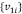, where i is associated to each vertex selected from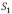, and an r radius neighborhood is established (a value of r=10 was chosen in this case) around. Figure 2 shows different selected neighborhoods.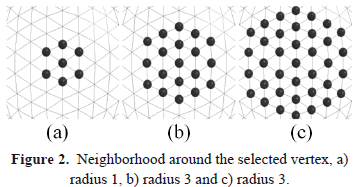From each neighborhood arounda histogram of the shape index is generated, and a search for neighborhoods having similar histograms is carried out in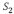. Figure 3 shows the result obtained from two types of range image. The foot corresponds to synthetic data and the frog is from real range data. The results reported in Table 1 shows the benchmark of the implemented tests. The proposed method shows an error value comparable with the other methods. However, the time used by the proposed method was lower. All measurements were obtained using a registration range data toolbox  implemented using Matlab software.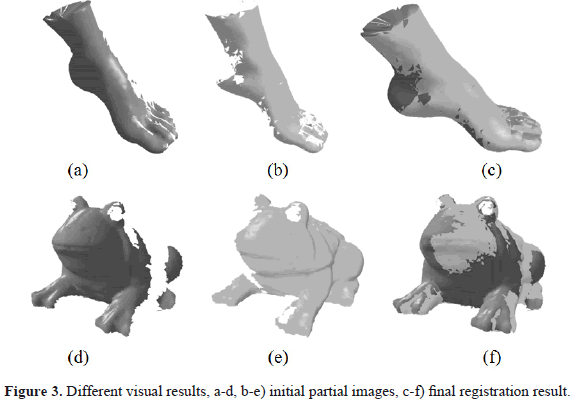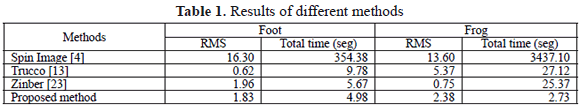Figures 4(a), 4(b) and 4(c) show the result of the registration process applied to the Stanford armadillo.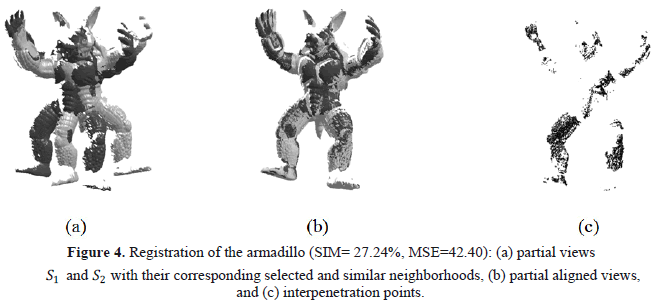5. CONCLUSIONS

In this work, a range image registration method is proposed, based on the geometric features described by the shape index per vertex.

This method is a starting point for the design of an automatic registration method, and presents some advantages such as its independence under rigid transformations. Furthermore, this method is deterministic, that is, with the same parameters the same result is obtained. One of its weaknesses is the fact that the result depends on the initial selection of points on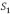; a bad selection will lead to an incorrect registration, besides, a more robust method is needed to detect false matches for neighborhoods having similar histograms but in different regions.

As future work, it is proposed to establish a selection criterion of initial points, so that unique histograms can be obtained, and establish a correspondence validation method by using Point Signature , with which the matching neighborhood structure can be compared. Additionally, the registration can be implemented with scale independence, so that if any of the partial views presents a different scale, this can be corrected when carrying out the alignment.

REFRENCES

 Branch J. W., Reconstrucción de objetos de forma libre a partir de imágenes de rango empleando una red de parches NURBS, Ph.D. dissertation, Universidad Nacional de Colombia, 2007.         [ Links ]
 Sanchez, G., Branch, J. y Boulanger, Pierre., Reconstrucción de objetos de topología arbitraria mediante selección de centros para la interpolación con FBR. Dyna: vol. 73, no. 150, pp. 189-201, 2006.         [ Links ]
 Chua, C. J. R., Point Signatures: a new representation for 3d object recognition, Int. J. Comput. Vision, vol. 25, (1), pp. 63-85, 1997.         [ Links ]
 Johnson, A., Spin-Images: A Representation for 3-D Surface Matching, Ph.D. dissertation, Robotics Institute, Carnegie Mellon University, Pittsburgh, PA, Aug. 1997.         [ Links ]
 Chung, D. and Lee, Y. D. S., Registration of multiple-range views using the reverse-calibration technique, Pattern Recogn., vol. 31, (4), pp. 457-464, 1998.         [ Links ]
 Song Chen, C., Ping Hung, Y. and Cheng. J., Ransac-based darces: A new approach to fast automatic registration of partially overlapping range images, IEEE Transactions on Pattern Analysis and Machine Intelligence, vol. 21, pp. 1229-1234, 1999.         [ Links ]
 Tarel, J., Civi, H. and Cooper, D., Pose estimation of free-form 3d objects without point matching using Algebraic Surface Models, in Proceedings of IEEE Work shop on Model-Based 3D, pp. 13-21. 1998.         [ Links ]
 Stamos, I. and Leordeanu, M., Automated feature-based range registration of urban scenes of large scale, IEEE Computer Society Conference on Computer Vision and Pattern Recognition, vol. 2, pp. 555-561, 2003.         [ Links ]
 Brunnstrom, K. and Stoddart, A., Genetic algorithms for free-form surface matching, in International Conference of Pattern Recognition, Vienna, pp. 689-693. 1996.         [ Links ]
 Feldmar, J. and Ayache, N., Rigid and affine registration of smooth surfaces using differential properties, in ECCV (II), pp. 397-406. 1994.         [ Links ]
 Faugeras, O., The Representation, Recognition, and Locating of 3-D Objects, Int. J. Robotic Res., vol. 5, (3), 1986.         [ Links ]
 Dorai, C., Weng, J. and Jain, A., Optimal Registration of Object Views Using Range Data, Trans. PAMI, vol. 19, (10), 1997.         [ Links ]
 Trucco, E., Fusiello, A. and Roberto, V., Robust motion and correspondences of noisy 3-D point sets with missing data, Pattern Recognition Letters, vol. 20, (9), pp. 889-898, Sep. 1999.         [ Links ]
 Rusinkiewicz. S., Estimating curvatures and their derivatives on triangle meshes, in Symposiumon 3D Data Processing, Visualization, and Transmission, Sep. 2004.         [ Links ]
 Koenderink, J. J. and Van Doorn, A. J., Surface shape and curvature scales, Image Vision Comput., vol. 10, (8), pp. 557-565, 1992.         [ Links ]
 Rubner, Y., Tomasi, C. and Guibas, L. J., A metric for distributions with applications to image databases, in ICCV '98: Proceedings of the Sixth International Conference on Computer Vision. Washington, DC, USA: IEEE Computer Society, P. 59. 1998.         [ Links ]
 Rachev, Themonge-Kantorovich. S. T., Mass transference problem and its stochastic applications, Theory of Probability and its Applications, vol. 29, (4), pp. 647-676, 1985.         [ Links ]
 Hitchcock, F. L., The distribution of a product from several sources to numerous localities, Journal of Math. Phys., vol. 20, pp. 224-230, 1941.         [ Links ]
 Pele O. and Werman, M., A linear time histogram metric for improved sift matching, in ECCV, 2008.         [ Links ]
 Silva, L., Bellon, O. and Boyer. K., Precision range image registration using a robust surface interpenetration measure and enhanced genetical gorithms, vol. 27 (5), pp. 762-776, May 2005.         [ Links ]
 Bentley, J. L., Multidimensional binary search trees used for associative searching, Commun. ACM, vol. 18 (9), pp. 509-517, September 1975.         [ Links ]
 Turk G., The Stanford bunny, 2000, [Online; accessed May 14, 2007]. [Online]. Available: http://www.gvu.gatech.edu/people/faculty/greg.turk/bunny/bunny.html         [ Links ]
 Zinber, T., Schmidt, J., Niemann, H., A refined ICP algorithm for robust 3D correspondence estimation. En: in IEEE International Conference on Image Processing, 2003.         [ Links ]
 Salvi, J., Matabosch C.: Range Image Registration Toolbox. - [Online; accessed Dic 10, 2010]         [ Links ].